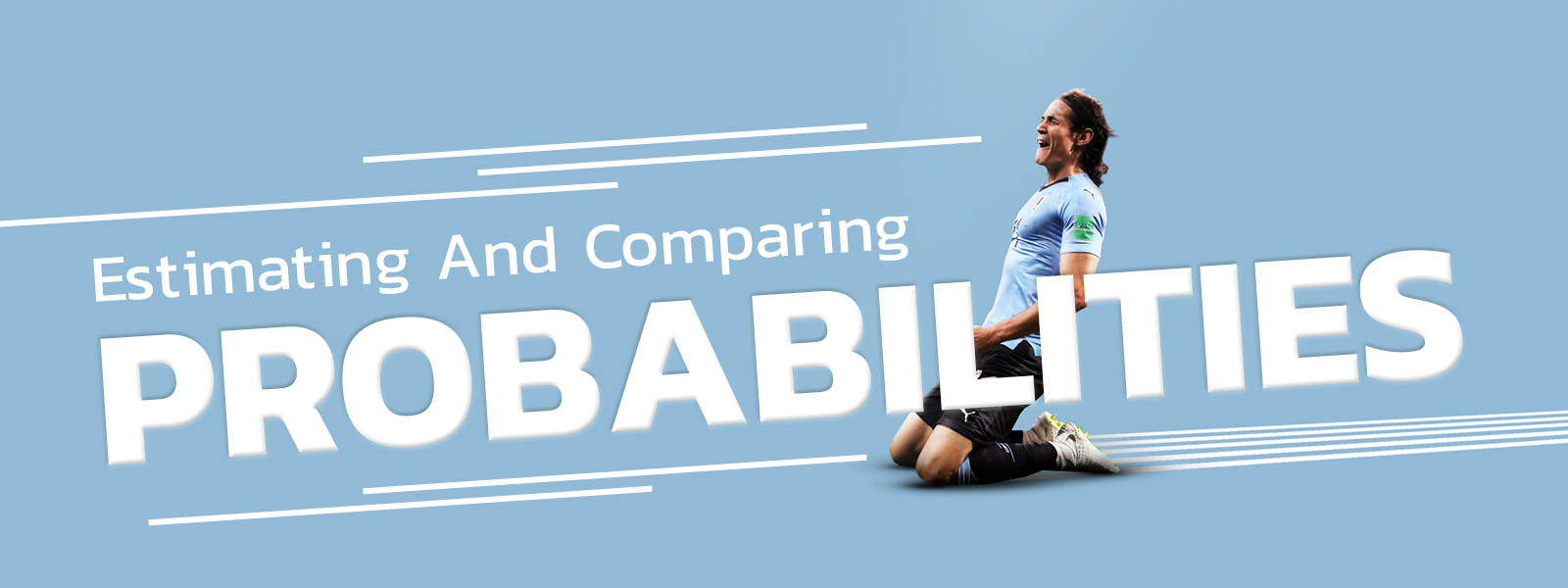Blog
Estimating And Comparing Betting Odds Probabilities
Written by, Jacob Cook Thu 25 Jun
Estimating And Comparing Betting Odds Probabilities## Comparing Betting Odds Probabilities

Betting odds pretty much function as the foundation of each bet you make. Firstly, they’re an indication of your potential profits. But, more importantly, they can be used to gauge the probability of an event occurring. There are several types of betting odds – fractional, decimal moneyline, and each of them requires a different approach to calculate the implied probability.

We know all of this can be quite overwhelming at first glance, which is why we have made this quick guide on how you can estimate and compare probabilities. Forget about in-depth analysis and strategies. The one thing you need to be able to do for your bet to be even remotely successful is to read odds. At first glance, especially to a beginner, all those numbers can be overwhelming and somewhat confusing. But once you get the hang of everything, odds and what they imply will become second nature.

Here’s how you estimate and compare probabilities using odds while betting.

## What is implied probability?

Implied probability is essentially the conversion of the betting odds into a percentage that represents the likelihood of that outcome occurring. Depending on the type of betting odds you’re dealing with, the method to calculate implied probability is slightly different. Let’s take a look at each of them.

### 1. Decimal odds

Decimal odds are perhaps the most popular of all the odds, especially in Europe, Australia, New Zealand, and Canada. Decimal odds represent the amount you would win if you bet \$1, or the country’s equivalent currency. Calculating the implied probability with decimal odds is quite straightforward. Here’s the formula you would follow: (1 ÷ odds) × 100.

Example:

• This is what the odds for an upcoming match between Norwich City and Arsenal look like - Norwich - 4.75, Draw – 4.3, Arsenal – 1.8
• To find Norwich’s implied probability, you would have to use the formula from above: (1 ÷ 4.75) × 100 = 21.1%.

Following the same formula, you would find that Arsenal has a 55.6% chance of winning, and a draw has a 23.3% possibility of occurring. As you can tell, the most likely outcome for this scenario is Arsenal winning, followed by a draw, and last, Norwich winning.

### 2. Fractional odds

Fractional odds are very popular in England. They tell you how much you will receive if you bet a particular amount. Here’s how you would calculate implied probability: (Denominator ÷ (numerator + denominator)) × 100

Example:

• The odds for a match between Newcastle and Manchester City are as follows - Newcastle – 18/1, Draw – 7/1, Manchester City - 1/5
• Let’s use the formula to calculate City’s implied probability:(5 ÷ (1+5)) × 100 = 83%.

If you were to do the same for Newcastle, you’d find that they have a 5.3% chance of winning, and the likelihood of a draw occurring is at 12.5%.

### 3. Moneyline odds

Moneyline odds are especially popular in North America and are expressed using a three-digit whole number. Calculating the implied probability is slightly different, as these odds are expressed in negative and positive values. Here’s the formula you would need to follow for a negative value: (- (negative moneyline odds)) ÷ (- (negative moneyline odds)) + 100. Positive moneyline odds have a slightly different, more simple method: 100 ÷ (positive moneyline odds + 100)

Example:

The odds for a match between Real Madrid and PSG. Real Madrid: -300, Draw: 500, PSG: 200

Let’s calculate Real’s implied probability first:

• (- (-300)) ÷ (- (-300) + 100) = 75%
• PSG’s implied probability would be as follows:
• 100 ÷ (200 + 100) = 33.3%

Since the draw option is a positive value, the implied probably would be 16.7%. This means that Real Madrid has the highest probability of winning.

recent post
categories
tags
Betting Articles